# Single Digit Addition

#### Excel add-in with c# VSTO and web

7 Lectures 1.5 hours

#### Word add-in using c# vsto and web types

8 Lectures 1 hours

#### The Ultimate Guide To Add Social Media Login to PHP Web Apps

17 Lectures 2 hours

Add the single digit numbers.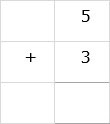### Solution

Method 1

Adding is just like counting in this method.

Step 1:

We pick a number to begin with; let’s say, 5.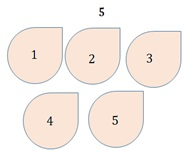Step 2:

We count up from the starting number (5) by the number of places as the second number (3) i.e., 6, 7 and 8 and we get the sum as 8.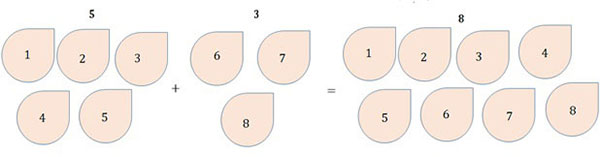Method 2

Step 1:

We can also use a number line to find the sum. We start from 5 on the number line and move three places to the right to land on 8. This is the sum.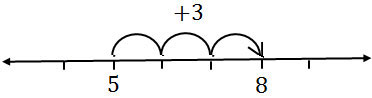Step 2:

SoAdd the single digit numbers.### Solution

Method 1

Step 1:

We start counting with the greater number 7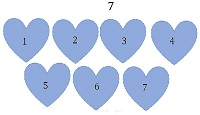Step 2:

And then we count up 4 more to get 11 as the sum.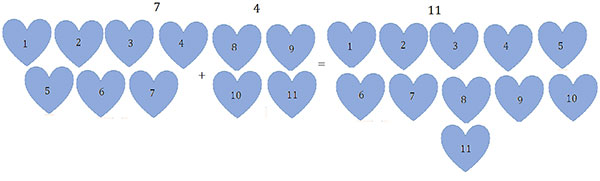Method 2

Step 1:

We can also use a number line to add numbers 7 and 4. We start from the greater number 7 on the number line and take 4 jumps to the right to land on the number 11 which is the sum.Step 2:

So